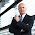## Forecasting package in R

Probably the last post before year end and very typical for me is to review this years system performance. A couple of R-packages are since 1 year the backbone of my lab. Just recently I added a package called "Forecast" which is a brilliant implementation of a couple of well known models. It is well explained in a free book accompanying this package https://www.otexts.org/fpp/ and explaining the math in detail.

To understand the differences of the forecast models better I wrote some code to backtest on the DAX and the DAX ETF (EXS1.DE) with some Parameters.

#-------------------------------------------------
#Parameters
#-------------------------------------------------
backval <- seq(20,50,by=5)
confidenceval<-c(.80,.95,.99)
sfcval=seq(.02,.06,by=.005)
confidence=confidenceval,threshold=sfcval)
#models <- list(m1=hw,m2=holt,m3=ses,m4=forecast,m5=naive,m6=meanf,m7=thetaf)
models <- list(m1=ets,m2=auto.arima,m3=hw,m4=holt,m5=thetaf,m6=naive)

stat <- matrix(nrow=nrow(para)*10+length(models),ncol=10)
colnames(stat)=c("z", "model","para","match", "long","profit","winner","loser","limit exit","limit wrong")
stat[,]=0

I've decided to take a history of up to 50 days(observations), which might be inappropriate for auto.arima which is not only a call to a model as the name suggests auto.arima returns also the optimal parameters found for the given period. That's why the code appears to be slow but there is a lot going on and it is definitely faster as my R-optimizer I used for finding the best model AIC as it is implemented in C++.

Once you have the xts object you can loop like that

xvars <- x[posab:posbis,c(4)]

for(y in 1:length(models)){

cfit=suppressWarnings(try(models[[y]](xvars),silent=T))

xvars will hold the back period and ahead is the prediction period.

I found the ets and the holt model as pretty good predictors but I'm still investigating.

That was it from me for 2013 have a merry Christmas and a wonderful 2014.

#### 1 comment :

1.I got various questions with regard to my comment above that the holt and ets models seems to be good predictors. I'm currently only using the predictions to optimize my money management aiming to get better stoplimit and profittargets and this seems to be promising - I'm not investigating entry signals or timing signals.